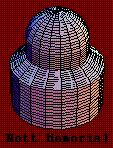WeBWorKUnion College
Department of Mathematics
WeBWorK Homework Server

WeBWorK courses for Spring term 2020:

 Math 102 - 1 Calculus with Precalculus III Prof. Khatami Math 112 - 1 Calculus II Prof. Khanmohammadi Math 115 - 1 & 2 Calculus III Prof. Klangwang Math 115 - 3 Calculus III Prof. Johnson Math 117 - 1 Calculus IV Prof. Jauregui Math 117 - 2 Calculus IV Prof. Friedman Math 117 - 3 Calculus IV Prof. Gasparovic Math 130 - 1 Ordinary Differential Equations Prof. Hatley Math 130 - 2 Ordinary Differential Equations Prof. Khanmohammadi IMP 121 - 1 Integrated Math and Physics II Prof. Wang

Courses from past terms
An Introduction to WeBWork for Students

Union College Department of Mathematics